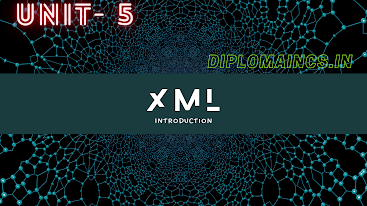## 8/28/19

### previous year question for computer science & engineering COMPUTER PROGRAMMING THROUGH C 2017

Computer Programming Through C

3rd Semester Diploma question of C Programming Language of year 2017 ODD

Sub Code: - 1600302

2017(Odd)
Time : 3Hrs
Sem. III(New)
CPTC(CSE)
Full Marks : 70
Pass marks : 28

Group A

Choose the most suitable answer from the following options: -   (1*20=20)

(i)  By default a function return a value of type
(a) int
(b) char
(c) void
(d) none of these

(ii) What will be the value of "x" after executing the program ?
void main()
{
int x;
x= printf("sbte");
printf ("x=%d" x);
}
(a)  x= 15
(b) x = 0
(c) garbage
(d) Error

(iii) Which of the following is not a relational operator ?
(a) !
(b) !=
(c) >=
(d) <

(iv) Which among the following is a unconditional control structure?
(a) Do-while
(b) If-else
(c) Goto
(d) For

(v) Does logical operator in C language are evaluated with short circuit?
(a) True
(b) False
(c) Depends on compiler
(d) None

(vi) Relational operator cannot be used on
(a) Structure
(b) Long
(c) String
(d) Float

(vii) Which of the following are a collection of different data-typed ?
(a) array
(b) Structure
(c) char
(d) All of the above

(viii) Which of the following symbol is used for condition checking?
(a) ♢
(b)
(c) 🗆
(d)  none

(ix) Who is the father of C language
(a) Charles Babage
(b) Dennis Ritchie
(c) Yaswant Kanetkar
(d) None of these

(x) C programme are converted into machine language with the help of
(a) an editor
(b) Compiler
(c) Operating system
(d) none

(xi) Standard ANSI C has __________ number of keywords?
(a) 30
(b) 32
(c)  24
(d) 36

(a) Number
(b) Special symbol
(c) Both (a) and (b)
(d)An alphabet

(xiii) Which of the following is a Bitwies operator
(a) &&
(b) }}
(c) !=
(d) &

(xiv) Which of the following can never be sent by call-by-value?
(a) variable
(b) Array
(c) Structure
(d) Both (b) & (c)

(xv) Select odd one out with respect to type?
(a) Char
(b) Int
(c) Long
(d) Float

(xvi) Which type of variable can have same name in different function
(a) Global variable
(b) Static Variable
(c) Function Argument
(d) Both (b) and (c)

(xvii) For 16-bit compile allowable range for integer constant __ is
(a) -3.Ge38 to 3.Ge38
(b) -32767 to 32768
(c) -32768 to 32767
(d) -32688 to 32667

(xviii) Which of the following is correct hierarchy of arithmetic operation in C
(a) / + * -
(b)  * - / +
(c) + - / *
(d) * / + -

(xix) Collection of similar data type is _____________
(a) Array
(b) Structure
(c) Vector
(d) None

(xx) Which operations are known as ternary operator?
(a) ::, ?
(b) &&
(c) ? :
(d) None of the above

### Group B ➥ Answer all Five Questions: -                         (5*4=20)   1. Define flow chart,flow chart symbol and its importance. OR Write a C program to check odd or even.  2. Write a C program to swap the value of the variable without using temporary variable.  OR  Write syntax of if-else statement with program.  3. Define array and show how to declare 2D array in C language. OR Write down  the difference between pointer and array.  4. Write a C program to check Armstrong number. OR   Write a C program to find largest of group of any number using array.   5. Explain arithmetic,relational operator in c. OR      What is an operator ? explain logical and assignment operator. Group C ➥ Answer all Five Questions: -                         (6*5=30)      6. Write a C program to read a matrix and print its transpose using array.                                                     OR Write a C program to addition of two matrix.   7.Write a program to test the given no. is prime or not.                                                      OR      Write a program to find GCD of two numbers using recursion.   8.Explain call by value and call by reference with suitable example.                                                       OR    Write a C program to show the following patter:                A               ABC              ABCDE             ABCDEFG            ABCDEFGHI   9. Explain switch statement with syntax and example.                                                       OR      Write a program in C to find the area of and perimeter of a circle using switch case.  10.Write a C program to calculate factorial and also check even or odd number using function.                                                        OR State the declaration syntax of pointer , and pointer initialization with example. _____________________________________________ (Note:- Update available soon, comment for any type of help) {Other links  COA : - https://diplomaforcse.blogspot.com/2019/08/previous-year-question-for-computer_42.html OS https://diplomaforcse.blogspot.com/2019/08/previous-year-question-for-computer_26.html ISP: - https://diplomaforcse.blogspot.com/2019/08/previous-year-question-for-computer_31.html NOTES of COA (unit-1): - https://diplomaforcse.blogspot.com/2019/09/notes-for-computer-science-engineering.html NOTES of ISP (unit-4): - https://diplomaforcse.blogspot.com/2019/09/notes-for-computer-science-engineering_21.html NOTES of OS (unit-1): - https://diplomaforcse.blogspot.com/2019/11/notes-for-computer-science-and.html NOTES of OS (unit-2): - https://diplomaforcse.blogspot.com/2019/11/notes-for-computer-science-and_20.html } FOLLOW US  ON FACEBOOK:- https://www.facebook.com/Diploma-students-Computer-engg-120180636037243/

1.2.